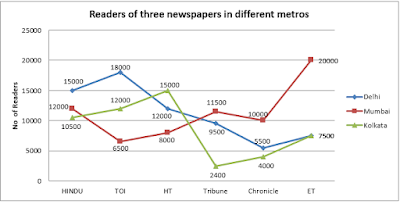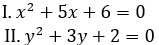# SBI Clerk Mains Quantitative Aptitude Quiz: 22nd JulySBI CLERK Quantitative Aptitude Quiz

Here, we are providing SBI CLERK Mains Study plan as there is left not enough time to deal in details. The questions asked in the quantitative aptitude section are calculative and very time-consuming. But once dealt with proper strategy, speed, and accuracy, this section can get you the maximum marks in the examination. Following is the Quantitative Aptitude quiz to help you practice with the best of latest pattern questions.

Q1. A pipe can fill a cistern in 12 min and another pipe can fill it in 15 min but a third pipe can empty it in 6 minutes. The first two pipes are kept open for 5 minutes in the beginning and then the third pipe is also opened. Time taken to empty the cistern is:

38 minutes
22 minutes
42 minutes
45 minutes
60 minutes

Q2. Taps A, B and C are attached with a tank and volumetric flow of water through them are 42 litre per hours, 56 litre per hours and 48 litre per hours, respectively. A and B are inlets and C is outlet. If all the taps are opened simultaneously, tank is filled in 16 hours. What is the capacity of the tank?

2346 litres
1600 litres
800 litres
960 litres
2330 litres

Q3. 8 men can finish a piece of work in 21 days. 14 men started working and after 3 days were replaced by 9 women. These 9 women finished the remaining work in 24 days. In how many days can days can 9 women finish the whole work?

24
26
36
32
30

Q4. A can do as much work as B and C together can do. A and B can together can do a piece of work in 9 hours 36 minutes and C can do it in 48 hours. The time (in hours) that B needs to do the work alone, is:

18 hrs
24 hrs
30 hrs
12 hrs
none of these

Q5. Two workers A and B are engaged to do a piece of work. A working alone would take 8 hours more to complete the work that when work together. If B worked alone, would take 4 ½ hours more than when work together. The time required to finish the work together is

5 hours
8 hours
4 hours
6 hours
none of these

Directions (6-10): Read the following graph and answer the questions that follow.Q6. What is the difference between TOI reader in Mumbai and Avg. of Tribune readers in all three Metros?

1350
1300
1750
1450
None of these

Q7. Total readers in Delhi except ET are approximately what percent of total readers in Mumbai except Chronicle and ET together?

140
150
165
160
170

Q8. How many readers are there in all three Metros for all newspaper?

186900
168900
189600
188600
None of these
Solution:

Delhi : 60000 + 7500 = 67500

Mumbai : 38000 + 30000 = 68000

Kolkata : 10500 + 12000 + 15000 + 2400 + 4000 + 7500 = 51400

Total users = 67500 + 68000 + 51400 = 186900

Q9. What is the ratio between one third of readers of Mumbai and three times the Hindu
Reader in all three metro together?

7 : 11
17 : 31
13 : 77
4 : 25
136 : 675

Q10. If total no of readers in Chennai are 30% less than in Kolkata but no of Tribune readers in Chennai are 25% more than the ET reader in Delhi, What is the total no of readers except Tribune in all four metro city now?

190015
109105
190105
180100
None of these

Directions (11-15): Two equations I and II are given below in each question. You have to solve these equations and give answer

Q11.if x < y
if x > y
if x ≤ y
if x ≥ y
if x = y or no relation can be established

if x < y
if x > y
if x ≤ y
if x ≥ y
if x = y or no relation can be established
if x < y
if x > y
if x ≤ y
if x ≥ y
if x = y or no relation can be established

if x < y
if x > y
if x ≤ y
if x ≥ y
if x = y or no relation can be established
if x < y
if x > y
if x ≤ y
if x ≥ y
if x = y or no relation can be established

You May also like to Read: